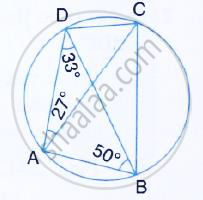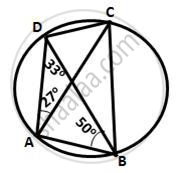Share

Abcd is a Cyclic Quadrilateral in Which ∠Dac = 27° ; ∠Dba = 50° and ∠Adb = 33°. Calculate: (I) ∠Dbc, (Ii) ∠Dcb, (Iii) ∠Cab - ICSE Class 10 - Mathematics

Question

ABCD is a cyclic quadrilateral in which  ∠DAC = 27° ; ∠DBA = 50° and ∠ADB = 33°. Calculate:
(i) ∠DBC, (ii) ∠DCB, (iii) ∠CABSolution(i) ∠DBC =∠DAC = 27°
(Angle subtended by the same chord on the circle are equal)

(ii) ∠ACB = ∠ADB = 33°
∠ACD = ∠ABD = 50°
(Angle subtended by the same chord on the circle are equal)
∴ ∠DCB = ∠ACD +∠ACB = 50° +33°+ 83°

(iii) ∠DAB +∠DCB = 180°
(Pair of opposite angles in a cyclic quadrilateral are supplementary)
⇒ 27 + ∠83° =180°
⇒ ∠CAB = 180° - 110° = 70°

Is there an error in this question or solution?
Solution Abcd is a Cyclic Quadrilateral in Which ∠Dac = 27° ; ∠Dba = 50° and ∠Adb = 33°. Calculate: (I) ∠Dbc, (Ii) ∠Dcb, (Iii) ∠Cab Concept: Cyclic Properties.
S